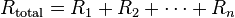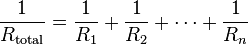# Resistance Equivalent

cjames9001

## Homework Statement

Find the equivalent resistance between points A and B shown in the figure . Consider R1 = 4.8 Ohms, R2 = 2.5 Ohms, R3 = 3.7 Ohms, R4 = 4.4 Ohms, R5 = 4.1 Ohms, and R6 = 6.4 Ohms.

## Homework Equations## The Attempt at a Solution

I got 9.33 by adding resistors 3,4,5 in parallel [1/(1/R3+1/R4+1/R5)] and added that to R6 which is in series with the equivalent of 3,4,5 and I added 1,2 In parallel [1/(1/R1+1/R2)]. My question is that obviously I am not combing the right resistors as parallel or series, can someone help me on this? I have also tried the equivalent of 3,4,5 in parallel and adding that to the equivalent of 1,2,6 in parallel which I believe is about 2.66 anyways I've tried a few other combinations as well and cannot seem to figure out which ones I am supposedto add in parallel and which ones to add in series

## The Attempt at a Solution

#### Attachments

Last edited by a moderator:

Mentor

First, you found an equivalent resistance for R's 3, 4, & 5 which are in parallel. Let's call that R345. What value did you get?

Then you added R345 in series with R6 and got 9.33Ω. Call that R3456. That seems a bit high to me.

Perhaps you should lay out your calculations here and we can see what's going on.

cjames9001
The 9.33 I Got was the final answer.

This is what I did:

First R345=[1/(1/R3+1/R4+1/R5)]=[1/0.7414]=1.349 Ohms

Then I took R6= 6.4 Ohms and added it to 1.349 to get 7.749 Ohms

Then I Got R12=[1/(1/R1+1/R2)]=[1/0.608]=1.644 Ohms

Lastly I added 1.644 Ohms to 7.749 And got 9.39 Actually, Sorry.

Mentor
The 9.33 I Got was the final answer.

This is what I did:

First R345=[1/(1/R3+1/R4+1/R5)]=[1/0.7414]=1.349 Ohms

Then I took R6= 6.4 Ohms and added it to 1.349 to get 7.749 Ohms

Then I Got R12=[1/(1/R1+1/R2)]=[1/0.608]=1.644 Ohms

Excellent up to here.

Lastly I added 1.644 Ohms to 7.749 And got 9.39 Actually, Sorry.

Oops. The last two resistors (R12 and R3456) are in parallel, not series.

Actually, the value for R3465 is in parallel with R1 and R2, so you could find the final result from there by doing the parallel calculation on the three.

cjames9001
Ok, That worked, I'm a little confused as to why getting R3456 Is Parallel to R12? and Why wouldn't getting R126 And Adding it In series to R345 doesn't work? Thanks for your help so far by the way

Mentor
Draw a picture with R1, R2, and R3456. You'll see that all three are in parallel (they all have their ends connected to node A and node B).

cjames9001
Ok I see what you mean, Thanks Again!